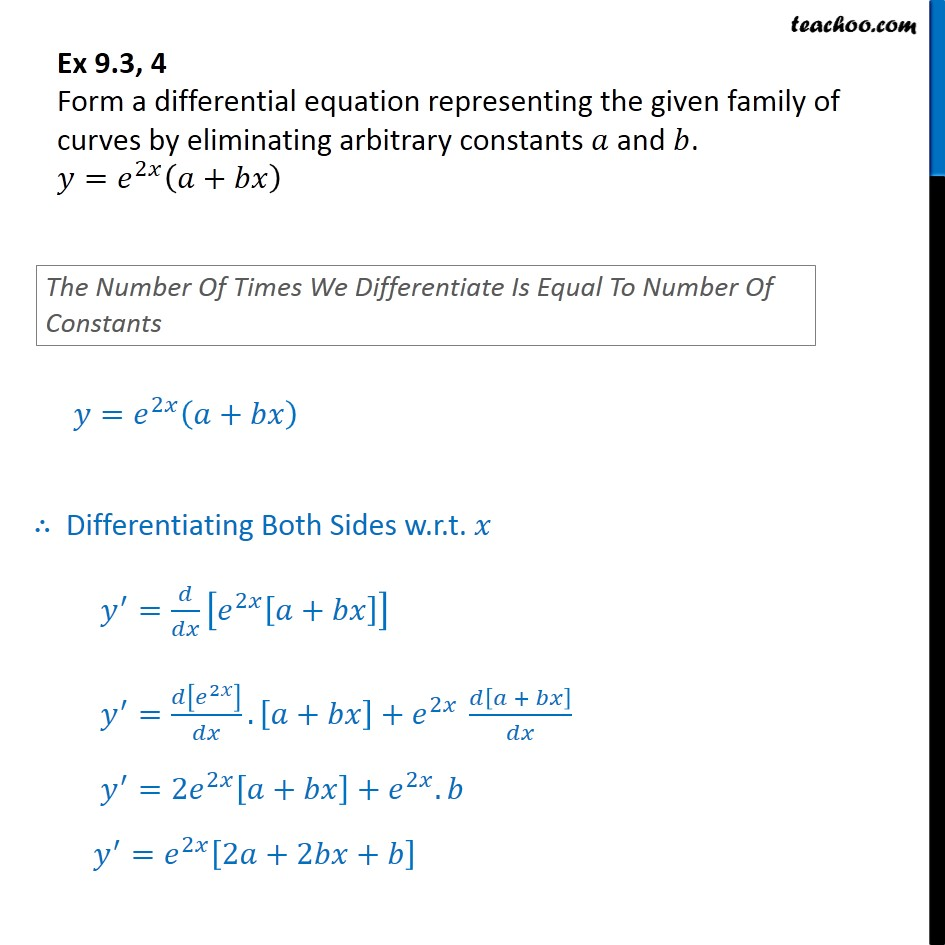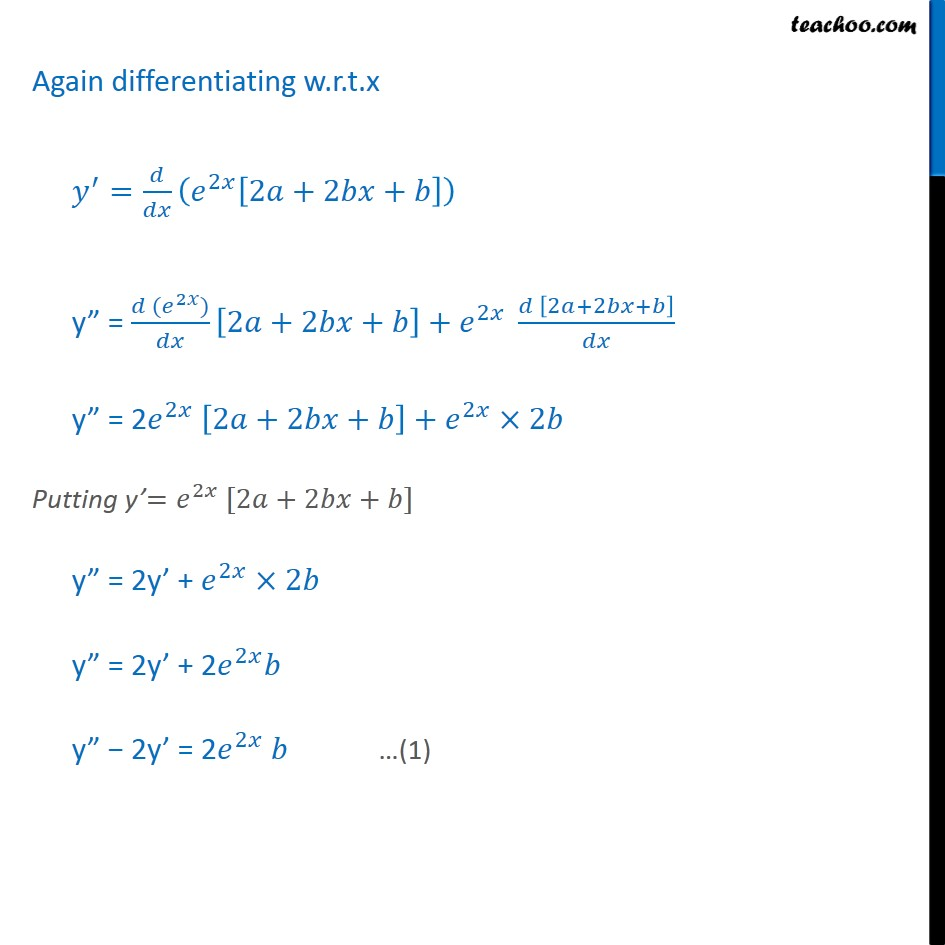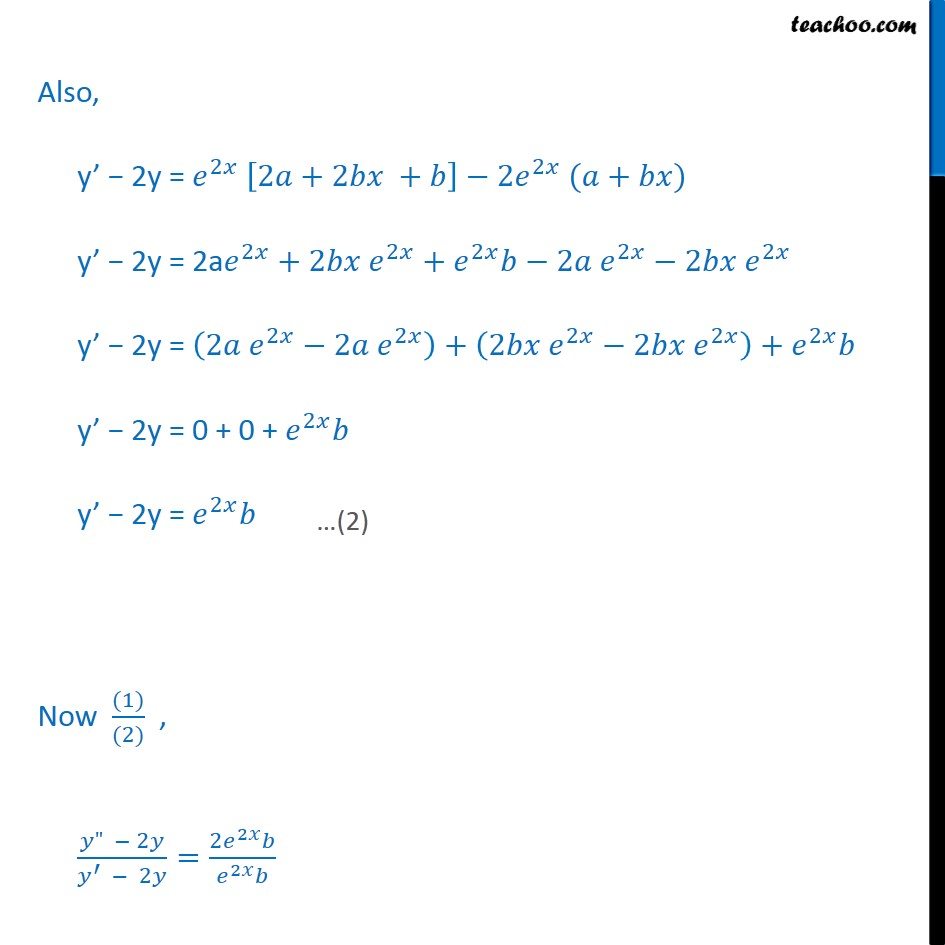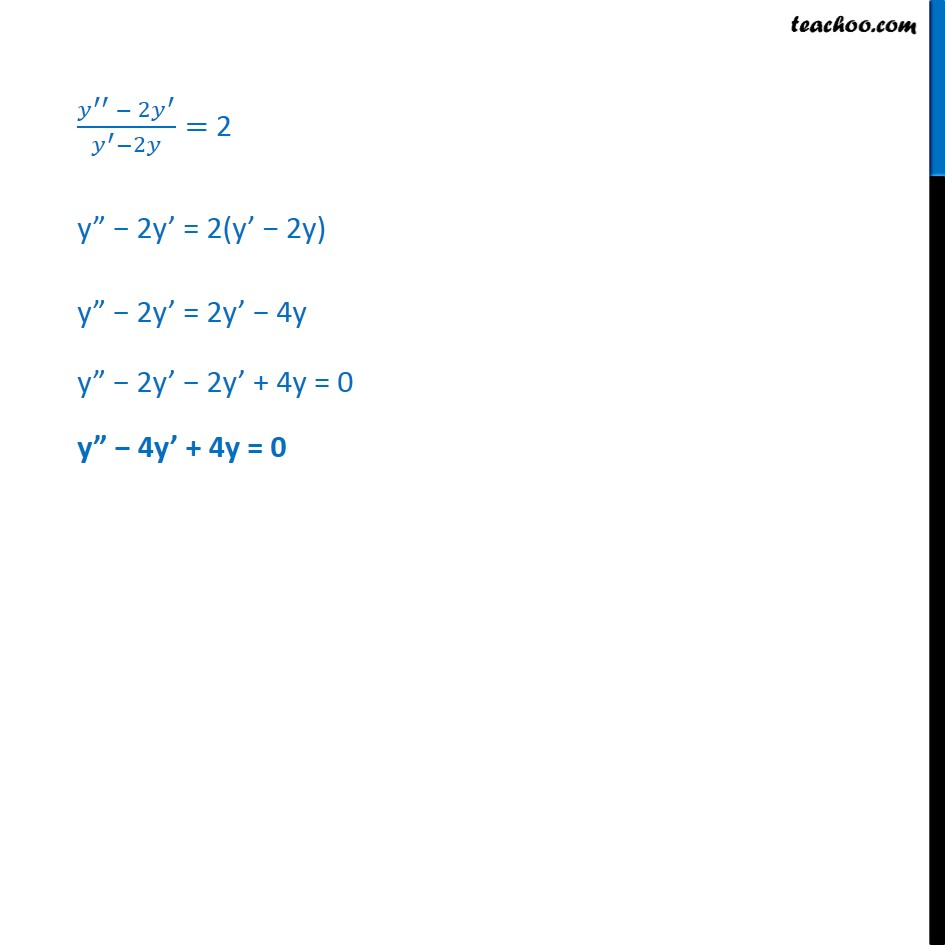Ex 9.3

Chapter 9 Class 12 Differential Equations
Serial order wiseGet live Maths 1-on-1 Classs - Class 6 to 12

### Transcript

Ex 9.3, 4 Form a differential equation representing the given family of curves by eliminating arbitrary constants 𝑎 and 𝑏. 𝑦=𝑒^2𝑥 (𝑎+𝑏𝑥) The Number Of Times We Differentiate Is Equal To Number Of Constants 𝑦=𝑒^2𝑥 (𝑎+𝑏𝑥) ∴ Differentiating Both Sides w.r.t. 𝑥 𝑦^′=𝑑/𝑑𝑥 [𝑒^2𝑥 [𝑎+𝑏𝑥]] 𝑦^′=𝑑[𝑒^2𝑥 ]/𝑑𝑥.[𝑎+𝑏𝑥]+𝑒^(2𝑥 ) 𝑑[𝑎 + 𝑏𝑥]/𝑑𝑥 𝑦^′=〖2𝑒〗^2𝑥 [𝑎+𝑏𝑥]+𝑒^2𝑥.𝑏 𝑦^′=𝑒^2𝑥 [2𝑎+2𝑏𝑥+𝑏] Again differentiating w.r.t.x 𝑦^′=𝑑/𝑑𝑥 (𝑒^2𝑥 [2𝑎+2𝑏𝑥+𝑏]) y” = (𝑑 (𝑒^2𝑥))/𝑑𝑥 [2𝑎+2𝑏𝑥+𝑏]+𝑒^2𝑥 (𝑑 [2𝑎+2𝑏𝑥+𝑏])/𝑑𝑥 y” = 2𝑒^2𝑥 [2𝑎+2𝑏𝑥+𝑏]+𝑒^2𝑥×2𝑏 Putting y’=𝑒^2𝑥 [2𝑎+2𝑏𝑥+𝑏] y” = 2y’ + 𝑒^2𝑥×2𝑏 y” = 2y’ + 2𝑒^2𝑥 𝑏 y” − 2y’ = 2𝑒^2𝑥 𝑏 Also, y’ − 2y = 𝑒^2𝑥 [2𝑎+2𝑏𝑥 +𝑏]−2𝑒^2𝑥 (𝑎+𝑏𝑥) y’ − 2y = 2a𝑒^2𝑥+2𝑏𝑥 𝑒^2𝑥+𝑒^2𝑥 𝑏−2𝑎〖 𝑒〗^2𝑥−2𝑏𝑥 𝑒^2𝑥 y’ − 2y = (2𝑎〖 𝑒〗^2𝑥−2𝑎〖 𝑒〗^2𝑥 )+(2𝑏𝑥 𝑒^2𝑥−2𝑏𝑥 𝑒^2𝑥 )+𝑒^2𝑥 𝑏 y’ − 2y = 0 + 0 + 𝑒^2𝑥 𝑏 y’ − 2y = 𝑒^2𝑥 𝑏 Now ((1))/((2)) , (𝑦" − 2𝑦)/(𝑦^(′ ) − 2𝑦)=(2𝑒^2𝑥 𝑏)/(𝑒^2𝑥 𝑏) (𝑦^′′ − 2𝑦^′)/(𝑦^′−2𝑦)= 2 y” − 2y’ = 2(y’ − 2y) y” − 2y’ = 2y’ − 4y y” − 2y’ − 2y’ + 4y = 0 y” − 4y’ + 4y = 0 (As 𝑦=𝑒^2𝑥 (𝑎+𝑏𝑥) ) Again differentiating w.r.t.x y” =𝑑/𝑑𝑥 (𝑒^2𝑥 [2𝑎+2𝑏𝑥+𝑏]) y” = (𝑑 (𝑒^2𝑥))/𝑑𝑥 [2𝑎+2𝑏𝑥+𝑏]+𝑒^2𝑥 (𝑑 [2𝑎+2𝑏𝑥+𝑏])/𝑑𝑥 y” = 2𝑒^2𝑥 [2𝑎+2𝑏𝑥+𝑏]+𝑒^2𝑥×2𝑏 Putting y’=𝑒^2𝑥 [2𝑎+2𝑏𝑥+𝑏] y” = 2y’ + 𝑒^2𝑥×2𝑏 y” = 2y’ + 2𝑒^2𝑥 𝑏 y” − 2y’ = 2𝑒^2𝑥 𝑏 …(1) Differentiating again w.r.t x 𝑦^′′−2𝑦^′=𝑑(𝑒^2𝑥.𝑏)/𝑑𝑥 𝑦^′′−2𝑦^′=2𝑒^2𝑥 𝑏 Dividing (1) and (2) i.e. ((2))/((1)) , (𝑦" − 2𝑦)/(𝑦^(′ ) − 2𝑦)=(2𝑒^2𝑥 𝑏)/(𝑒^2𝑥 𝑏) (𝑦^′′ − 2𝑦^′)/(𝑦^′−2𝑦)= 2 y” − 2y’ = 2(y’ − 2y) …(2) y” − 2y’ = 2y’ − 4y y” − 2y’ − 2y’ + 4y = 0 y” − 4y’ + 4y = 0 is the required equation